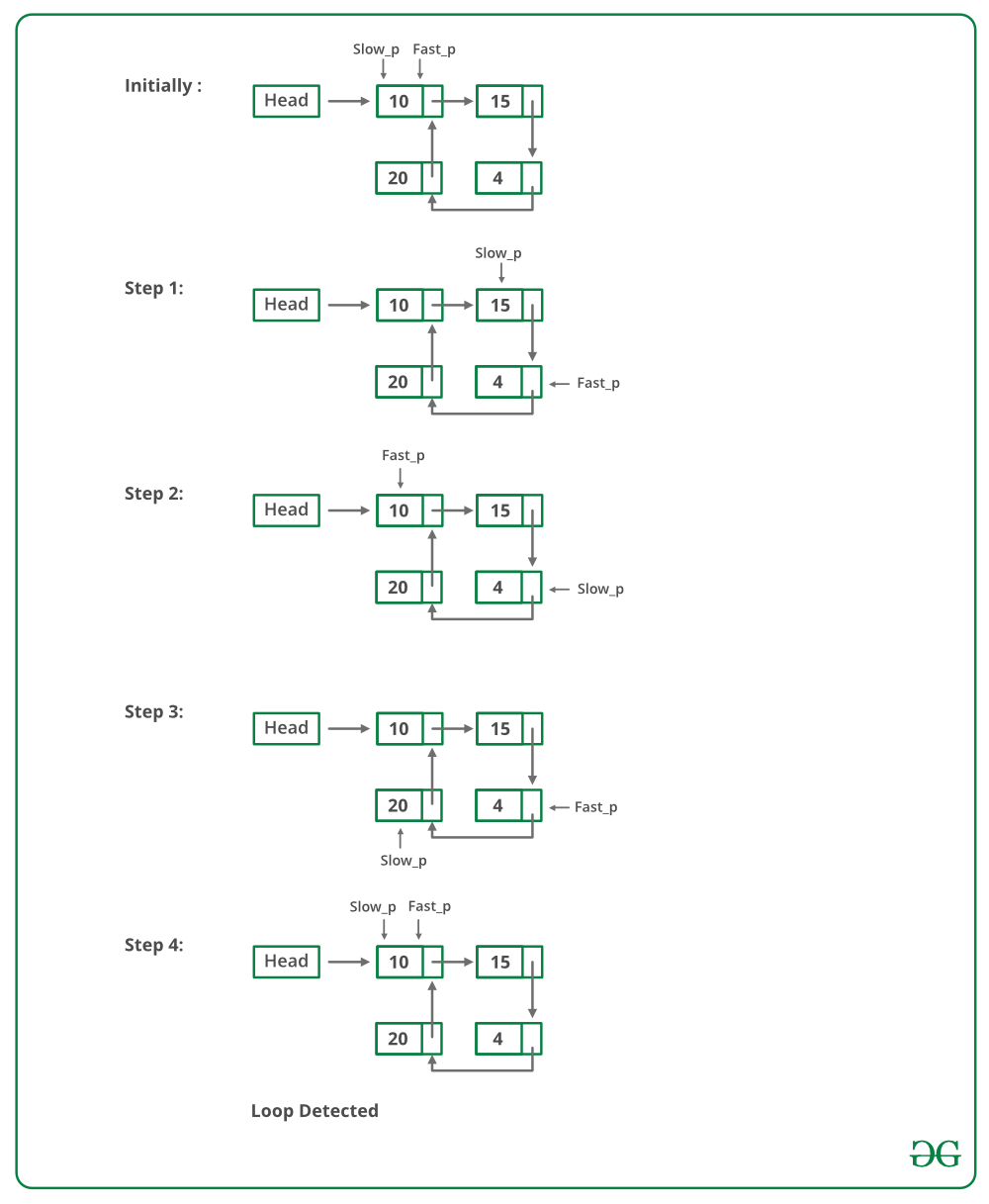Open in App
Not now

# C Program For Detecting Loop In A Linked List

• Last Updated : 11 Dec, 2021

Given a linked list, check if the linked list has loop or not. Below diagram shows a linked list with a loop.Solution: Floyd’s Cycle-Finding Algorithm
Approach: This is the fastest method and has been described below:

• Traverse linked list using two pointers.
• Move one pointer(slow_p) by one and another pointer(fast_p) by two.
• If these pointers meet at the same node then there is a loop. If pointers do not meet then linked list doesn’t have a loop.

The below image shows how the detectloop function works in the code:Implementation of Floyd’s Cycle-Finding Algorithm:

## C

 `// C program to detect loop in a linked list ` `#include ` `#include ` ` `  `/* Link list node */` `struct` `Node { ` `    ``int` `data; ` `    ``struct` `Node* next; ` `}; ` ` `  `void` `push(``struct` `Node** head_ref, ``int` `new_data) ` `{ ` `    ``/* allocate node */` `    ``struct` `Node* new_node ` `        ``= (``struct` `Node*)``malloc``(``sizeof``(``struct` `Node)); ` ` `  `    ``/* put in the data  */` `    ``new_node->data = new_data; ` ` `  `    ``/* link the old list off the new node */` `    ``new_node->next = (*head_ref); ` ` `  `    ``/* move the head to point to the new node */` `    ``(*head_ref) = new_node; ` `} ` ` `  `int` `detectLoop(``struct` `Node* list) ` `{ ` `    ``struct` `Node *slow_p = list, *fast_p = list; ` ` `  `    ``while` `(slow_p && fast_p && fast_p->next) { ` `        ``slow_p = slow_p->next; ` `        ``fast_p = fast_p->next->next; ` `        ``if` `(slow_p == fast_p) { ` `            ``return` `1; ` `        ``} ` `    ``} ` `    ``return` `0; ` `} ` ` `  `/* Driver program to test above function*/` `int` `main() ` `{ ` `    ``/* Start with the empty list */` `    ``struct` `Node* head = NULL; ` ` `  `    ``push(&head, 20); ` `    ``push(&head, 4); ` `    ``push(&head, 15); ` `    ``push(&head, 10); ` ` `  `    ``/* Create a loop for testing */` `    ``head->next->next->next->next = head; ` ` `  `    ``if` `(detectLoop(head)) ` `        ``printf``(``"Loop found"``); ` `    ``else` `        ``printf``(``"No Loop"``); ` `    ``return` `0; ` `}`

Output

`Loop found`

Complexity Analysis:

• Time complexity: O(n).
Only one traversal of the loop is needed.
• Auxiliary Space:O(1).
There is no space required.

How does above algorithm work?
Please See : How does Floyd’s slow and fast pointers approach work?